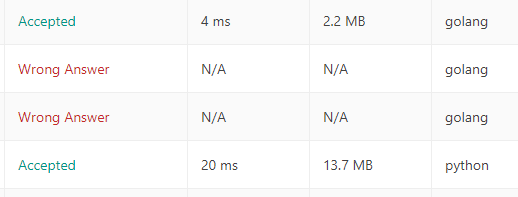# I tried LeetCode every day 7. Reverse Integer (Python, Go)

## Introduction

@Ishishow is running a free English word site E-tan.

I would like to work on letcode every day to improve my ability as a programmer and give my own way of solving it.

#### What is Leetcode

leetcode.com This is the practice of coding interviews for software developers. A total of more than 1,500 coding questions have been posted, and it seems that the same questions are often asked in actual interviews.

Introduction to Go language + algorithm I will solve it with Golang and Python to strengthen my brain. (Python is weak but experienced)

#### Day 2 (Problem 07)

Reverse Integer

--Problem content (Japanese translation)

If you specify a 32-bit signed integer, it is the reverse digit of the integer.

** Note: ** Assuming we are dealing with an environment that can store integers in the range of 32-bit signed integers: [-2 31, 2 31-1]. For the purposes of this problem, suppose the function returns 0 when the inverse integer overflows.

Example 1:

``````  Input: x = 123
Output: 321
``````

Example 2:

``````  Input: x = -123
Output: -321
``````

Example 3:

``````  Input: x = 120
Output: 21
``````

Example 4:

``````  Input: x = 0
Output: 0
``````

#### Way of thinking

1. Make a positive / negative judgment, and if it is negative, make it positive.
2. Reverse the numbers by loop processing
3. Describe the processing of INTMAX and INTMIN. (Overflow processing)

#### Explanation

1. I just applied a minus.
2. Assign the remainder after dividing by 10 to a variable called ans → and multiply ans by 10 before the next assignment (the reverse is true this way!)
3. For Go, I tried using the math package!

``````  class Solution(object):
def reverse(self, x):
if x < 0:
return (self.roop_func(-x) * -1)
else:
return self.roop_func(x)

def roop_func(self,x):
ans = 0
while x > 0:
ans = ans*10 + x % 10
x /= 10
if -(ans) < -(2**31) or ans > 2**31 - 1:
return 0
return ans
``````

--I'll write it in Go too!

``````  import "math"

func reverse(x int) int {
if x < 0 {
return -(roop_int(-x))
} else {
return roop_int(x)
}
}

func roop_int(x int) int {
ans := 0
for x > 0 {
ans = ans*10 + x%10
x /= 10
}
if -(ans) < math.MinInt32 || ans > math.MaxInt32 {
return 0
}
return ans
}
``````

Go and Python execution time

From the left, RunTime, Memory, language. Don't worry about making a mistake.### Another solution

There seems to be a way to solve it by reversing the characters. This person also worked on it, but it's easy. ..

``````class Solution(object):
def reverse(self, x):
s = (x > 0) - (x < 0)
r = int(str(x*s)[::-1])
return s*r * (r < 2**31)
``````

String manipulation is easy with python!

--Self memo (Go)

Go fails to compile if there are unused variables or packages

String cannot be nil

### Referenced articles

[Beginner] Pit for those who are new to Go, solutions to problems and common mistakes